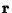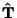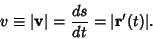## Velocitywhereis the Position Vector andis the derivative with respect to time. Expressed in terms of the Arc Length,whereis the unit Tangent Vector, so the Speed (which is the magnitude of the velocity) is